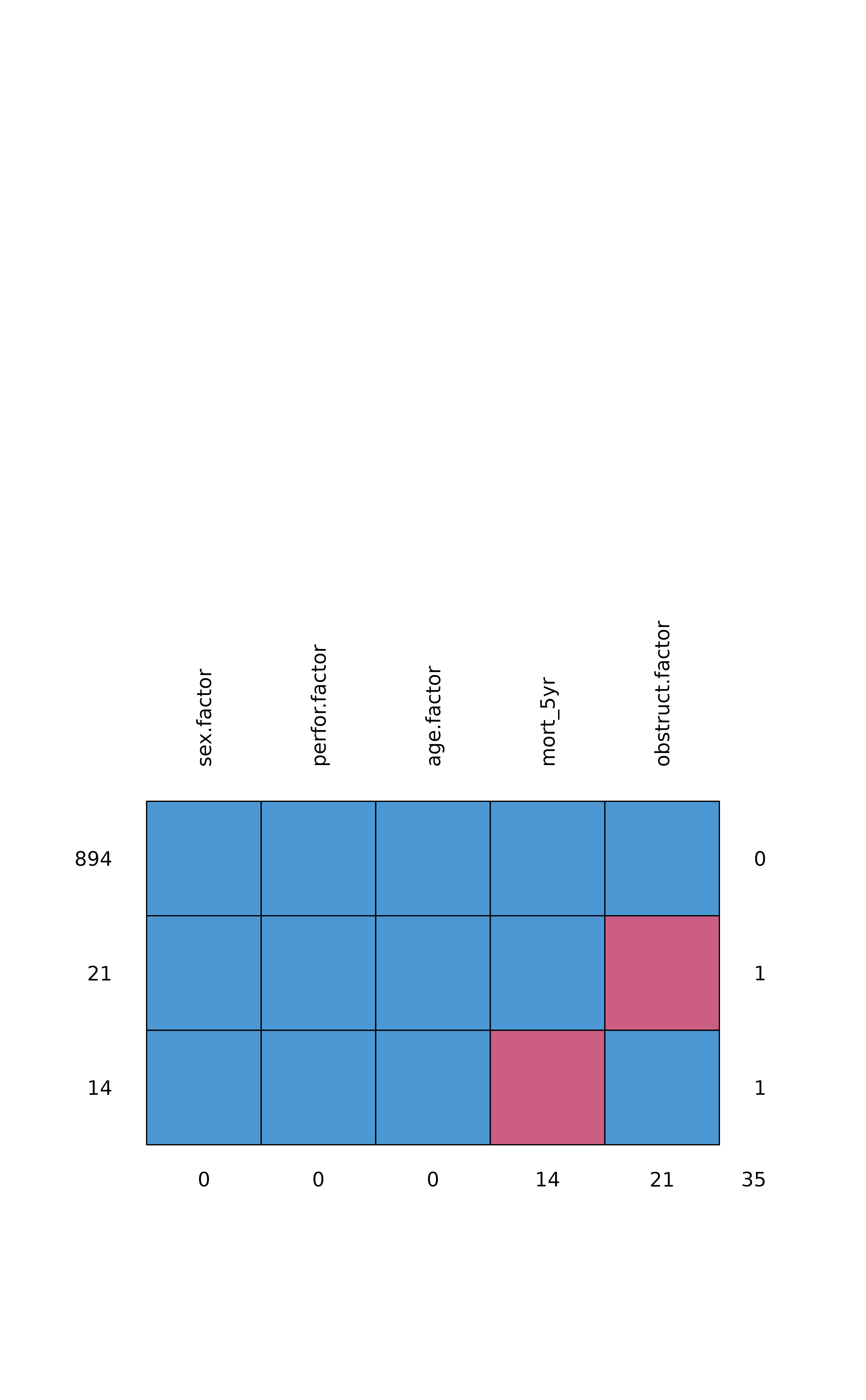Using finalfit conventions, produces a missing data matrix using md.pattern.

missing_pattern(
.data,
dependent = NULL,
explanatory = NULL,
rotate.names = TRUE,
...
)

## Arguments

.data

Data frame. Missing values must be coded NA.

dependent

Character vector usually of length 1, name of depdendent variable.

explanatory

Character vector of any length: name(s) of explanatory variables.

rotate.names

Logical. Should the orientation of variable names on plot should be vertical.

...

pass other arguments such as plot = TRUE to md.pattern.

## Value

A matrix with ncol(x)+1 columns, in which each row corresponds to a missing data pattern (1=observed, 0=missing). Rows and columns are sorted in increasing amounts of missing information. The last column and row contain row and column counts, respectively.

## Examples

library(finalfit)
library(dplyr)
explanatory = c("age.factor", "sex.factor", "obstruct.factor", "perfor.factor")
dependent = "mort_5yr"

colon_s %>%
missing_pattern(dependent, explanatory)#>     sex.factor perfor.factor age.factor mort_5yr obstruct.factor
#> 894          1             1          1        1               1  0
#> 21           1             1          1        1               0  1
#> 14           1             1          1        0               1  1
#>              0             0          0       14              21 35